Vibroengineering PROCEDIA

Published: 19 October 2018

# Sensitivity analysis of leaf blower vibration isolator based on ISIGHT

Wenbin Yu1
Beibei Sun2
Chuang Li3
1, 2, 3Southeast University, Nanjing, China
Corresponding Author:
Beibei Sun
Views 217

#### Abstract

In order to reduce the optimal design space of the vibration isolator, a sensitivity analysis method based on ISIGHT for the leaf blower vibration isolator was proposed in this paper. Parametric modeling of the isolator was realized by Solidworks, Hypermesh, Optistruct and ADAMS, and a multi-software co-simulation working framework based on ISIGHT was established. Taking the design parameters of the vibration isolator as the variable and the minimum transmission force as the optimization, Sensitivity analysis of the vibration isolator was carried out based on the Latin Hypercube. The results show that the length of the vibration isolator has the greatest influence on the vibration isolation and the contribution of d1, d3, D3 and E3 is the smallest. The result provided a guiding significance for the design optimization of the vibration isolator.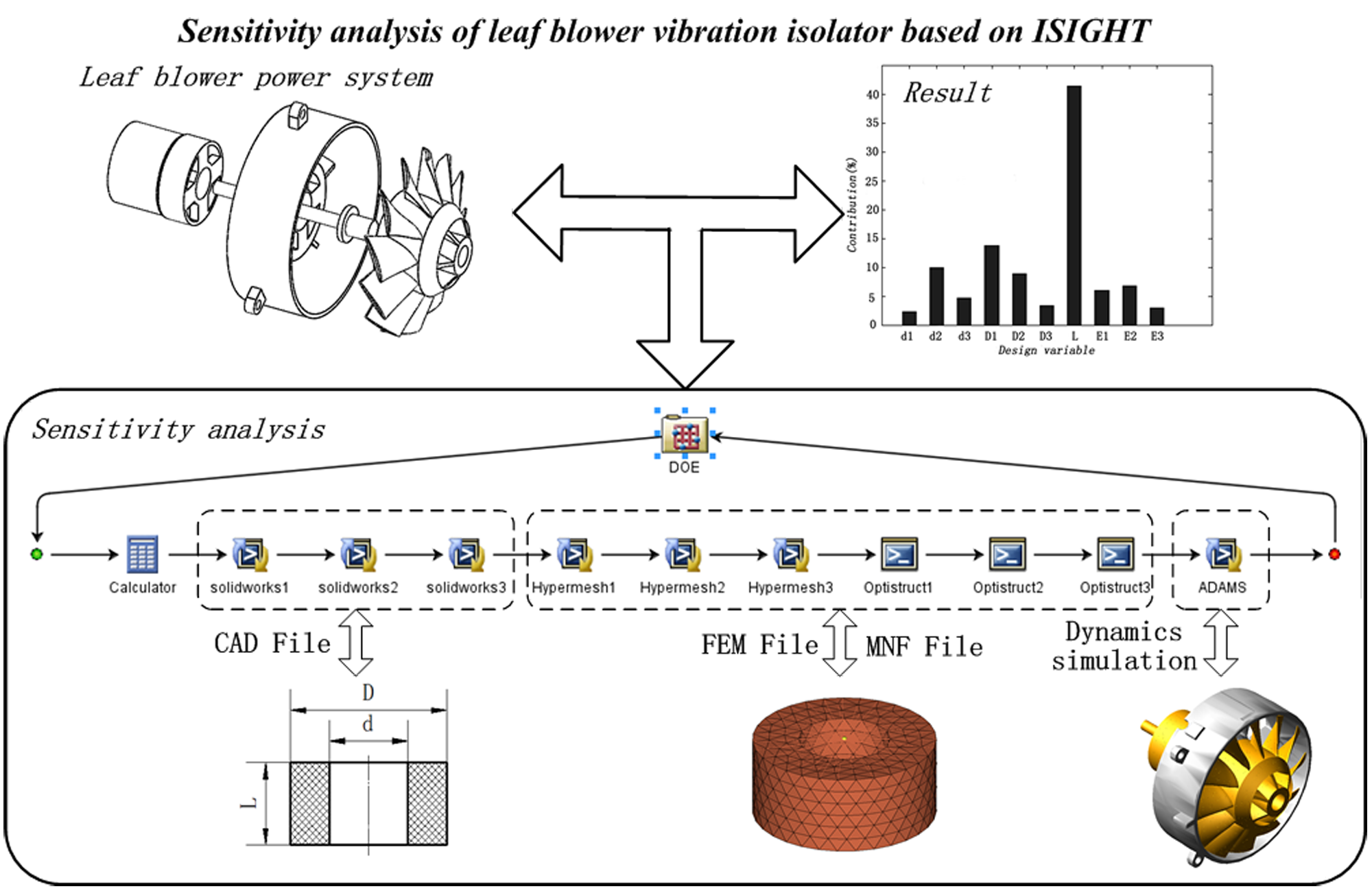#### Highlights

• A multi-software co-simulation working framework is established for dynamics simulation based on ISIGHT.
• Using the Latin Hypercube method to do sensitivity analysis of the vibration isolator.
• Sensitivity analysis is based on the parameters of the vibration isolator as variables and the minimum transmission force as optimization.

## 1. Introduction

The leaf blower vibration isolation effect is mainly determined by the stiffness of the isolator, the installation position, etc. as well as the stiffness of the isolator depends on the structure of the isolator and the material properties. Therefore, there are a large number of parameters in the optimization design process of the vibration isolator structure. If all the parameters are taken as optimization design variables, the calculation amount is very large. Through the sensitivity analysis, it can be determined which design parameters are most effective, so as to select as few optimization variables as possible and reduce the calculation amount of the optimized design . In recent years, scholars have proposed a large number of design theories and methods in the field of vibration isolation system. The design process generally calculates the theoretical stiffness of the isolator that meets the vibration isolation requirements of the system, and then designs and verifies the isolator according to the stiffness. This design method is cumbersome. Taking the vibration isolator structure and material parameters as design variables, taking the transmission force as the target is more convenient [2-6]. This method tends to bring more design variables. Therefore, for the multivariable optimization problem of the vibration isolation system, sensitivity analysis can determine which design parameters are most effective, which can greatly simplify the optimization design process. It is of great significance for the optimization of the vibration isolation system .

In this paper, the size parameters of the vibration isolator and the elastic modulus are taken as the design variables, the minimum transmission force of the power system to the housing is taken as the optimization. Based on ISIGHT software, multiple software is integrated to realize multi-software co-simulation. The Latin hypercube test method is used to analyze the sensitivity of the design variables to determine the influence of each design variable on the vibration isolation effect, thereby reducing the design space and improving the design efficiency.

## 2. Integrated system framework based on ISIGHT

ISIGHT is a software that drives product optimization design through software collaboration. Its function can be summarized as an automation integrated design optimization process. The key point to automating the optimization process is to implement reconstruction CAD model by parametric drive and simulate through batch mode. This paper combines the technical requirements of sensitivity analysis with rubber vibration isolator and builds an integrated framework based on ISIGHT. As shown in Fig. 1, batch files were created based on the parametric modeling features of each design software. Using the commercial software ISIGHT to call these batch files to realize the integration and collaboration of Solidworks, Hypermesh, Optistruct and ADAMS. Firstly, the dynamic model of the leaf blower power system was established based on ADAMS. Then, the vibration isolator geometry model was created for Hypermesh by Solidworks, a finite element model was created for Optistruct by Hypermesh. Furthermore, the isolator flexible body file was exported to the ADAMS by Optistruct. Finally, the dynamics solution was performed in the ADAMS.

Fig. 1Integrated modeling and collaborative simulation flow chart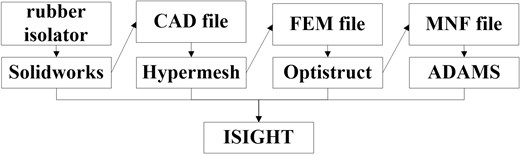### 2.1. The dynamic model of leaf blower power system

The power system of the leaf blower is shown in Fig. 2. The power system can be generally divided into five parts: fan, shaft, motor rotor, motor stator and collector. The rotor and the shaft of the motor are installed by interference fit. The fan and the shaft are fixed by bolts. The stator and the shaft are supported by two deep groove ball bearings. The stator and collectors are fixed by bolts. The collector and the housing are fixed through three bolts without any vibration isolation measures. Since the transmission force of the power system to the housing is studied in this paper and the mass of the blower housing is much greater than the power system, the housing can be regarded as a rigid foundation. It is known that the rotational speed of the blower is 17000 rpm. The stiffness of the shaft has a great influence on the dynamic response under high-speed rotation so that the shaft is built as a flexible body to establish a rigid-flexible coupling model of the power system. In this paper, the rigid-flexible coupling model of the power system was built by ADAMS and Hypermesh. The material properties of each component of the power system are shown in Table 1.

Fig. 2Structure and dynamic model of leaf blower power system where: 1 – bolt hole, 2 – motor, 3 – collector, 4 – shaft, 5 – fan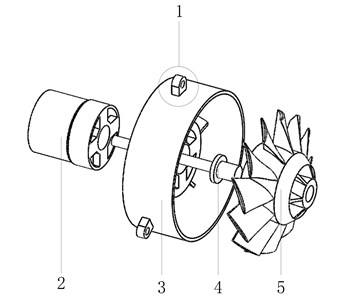a)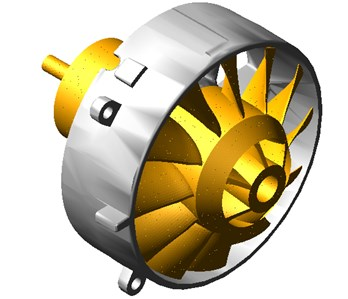b)

Table 1Material properties of components

 Part name Density (g/cm3) Elastic modulus (GPa) Shaft 7.85 210 Fan 1.35 / Rotor 7.85 / Stator 7.85 / Collector 2.70 /

### 2.2. Parametric modeling of rubber isolator

The original blower power system is fixed to the casing by bolts and the vibration is not effectively isolated. Because the rubber isolator can be designed into various shapes and different hardness, it is widely used in mechanical products. The basic shape of the isolator used in this paper is shown in Fig. 3. The rubber isolator structure is modeled by the Solidworks parametric modeling method. The characteristic sizes of the control shape are inner diameter $d$, outer diameter $D$ and length $L$.

Fig. 3Size parameters and finite element model of rubber vibration isolator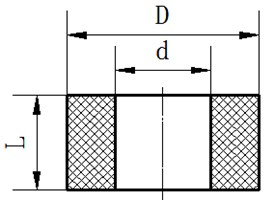a)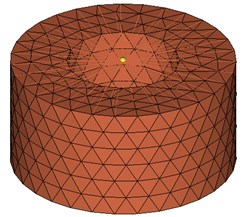b)

The rubber material has a nonlinear characteristic in the case of large deformation but can be considered to be linear in the case of small deformation. Because there is little pressure on the rubber isolator of the blade power system, it can be assumed that the stiffness of the isolator is linear. In this paper, the Command file is used to record the steps in Hypermesh, the steps including importing 3D geometry files, meshing, creating rubber isolator materials and assigning properties, creating attachment points and creating load steps. The complex process of manual modeling can be introduced into parametric modeling by transforming the code in the Command file. In this paper, Optistruct is used to solve the first ten order CB modes of rubber isolators. The parametric modeling method in Optistruct is the same as the method in Hypermesh.

The ADAMS macro command is a set of command sets that can execute a series of ADAMS/View commands. When creating a macro command, the list of executed ADAMS/View commands need to be listed firstly and then write them as macro commands. There are three ways to create macro commands in ADAMS: recording mode, editing and creating macro commands with the macro editor and creating macro commands by importing files. In this paper, the first method is used to create an ADAMS macro command. The specific implementation process is as follows:

Step 1: Enable the macro command recording function in ADAMS;

Step 2: Complete the tasks required in ADAMS: open the dynamics model of the blower power system, introduce the MNF file of the isolator, create fixed pairs between the isolator and the ground, create fixed pairs between the isolator and the power system, create simulation script, set result output;

Step 3: Terminate the recording of macro commands in ADAMS, open the macro command file, and extract the various command segments for executing the task.

## 3. Sensitivity analysis of vibration isolator structure

### 3.1. Optimized mathematical model

(1) Optimization.

In order to minimize the force transmitted by the power system to the blower housing. In this paper, the minimum total transmission force is used as the optimization in sensitivity analysis. The total transmission force can be expressed by Eq. (1):

1
$Min\left(F\left(x\right)\right)=\sum _{i=1}^{n}\sqrt{{{{f}_{xi}^{2}+f}_{yi}^{2}+f}_{zi}^{2}}.$

Here ${f}_{xi}$, ${f}_{yi}$, ${f}_{zi}$ represent the reaction force of the $i$th ($i=$ 1, 2, 3) rubber isolator in $x$, $y$ and $z$ directions.

(2) Design variable.

Since the stiffness of the isolator is mainly related to the material and size parameters of the isolator, the inner diameter $d$, the outer diameter $D$, the length $L$ and the elastic modulus $E$ of the three ring-shaped rubbers are taken as design variables. The value range of the design variables is shown in Table 2.

Table 2The range of design variable

 – ${d}_{i}$ (mm) ${D}_{i}$ (mm) ${L}_{i}$ (mm) ${E}_{i}$ (MPa) Initial value 4 10 5 2 Minimum value 2 6 2 1.5 Maximum value 5 12 10 5

(3) Constraint.

Since the vibration isolation rubber of blower power system is installed on the same plane, it is necessary to ensure that the length of the three rubber isolators is consistent. Considering the principle of vibration isolation, the smaller the stiffness of the brace, the better the vibration isolation effect is. However, the smaller the stiffness, the larger the amplitude of the power system will be. On the other hand, the greater the support stiffness, the smaller the amplitude, but the vibration isolation effect is poor. Taking into account the above constraints, the constraint expression is as follows:

2

Here $e$ is the amplitude of the centroid of the power system.

### 3.2. Sensitivity analysis

In order to analyze the influence of the structural design variables of the rubber isolator on the transmission force, the DOE was carried out in this section. In this paper, the Latin hypercube method was used to sampling based on the ISIGHT integration program built in the last section (Fig. 4).

The Pareto diagram is the histogram of the normalization coefficient of the factor, which indicates the influence on the result. Due to the large number of isolator parameters, the contribution of isolator parameters to the target value can be obtained by Pareto diagram (Fig. 5). The largest contribution is the length $L$ of the isolator, the contribution rate is about 42 %. The contribution rates of d1, d3, D3 and E3 are the smallest.

Fig. 4Sampling work flow chart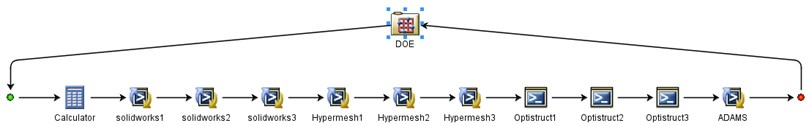Fig. 5The contribution of the vibration isolator parameters to the optimization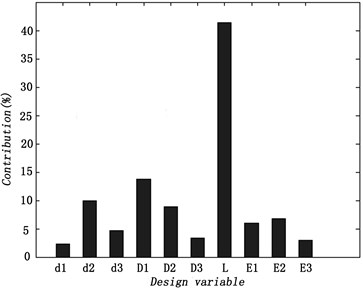## 4. Conclusions

Combined with the design parameters of the vibration isolator, parametric modeling of vibration isolator was realized by using multiple software. A multi-software co-simulation work framework was established based on ISIGHT. The sensitivity analysis of the vibration isolator is carried out by using Latin hypercube based on the simulation workflow. The results show that the length of the vibration isolator has the greatest influence on the vibration isolation and the contribution of d1, d3, D3 and E3 is the smallest. It is significant for the design optimization of the vibration isolator. The results of the sensitivity analysis can guide the design direction of the isolator and reduce design space for post-optimal design processes.